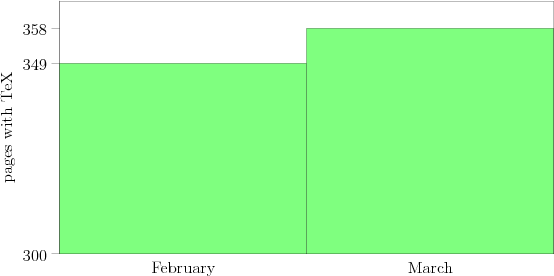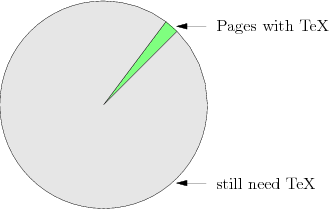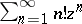# User:Nikita2

I am Nikita Evseev, a math graduate student living in Novosibirsk, Russia. My research interests are in Analysis and Sobolev spaces.

## TeXing

I'm keen on turning up articles of EoM into better appearance by rewriting formulas and math symbols in TeX.

Now there are 356 (out of 15,890) articles with Category:TeX done tag.$\quad \rightarrow \quad$ $\sum_{n=1}^{\infty}n!z^n$ Just type $\sum_{n=1}^{\infty}n!z^n$. Today You may look at Category:TeX wanted.

How to Cite This Entry:
Nikita2. Encyclopedia of Mathematics. URL: http://www.encyclopediaofmath.org/index.php?title=Nikita2&oldid=29534